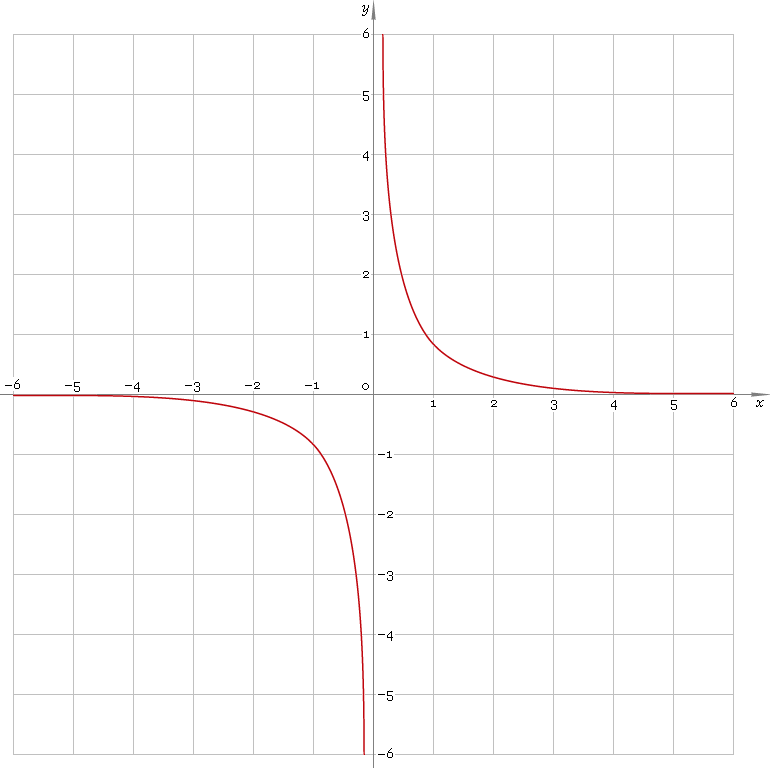The Art of Interface

# csch — hyperbolic cosecant function

Category. Mathematics.

Abstract. Hyperbolic cosecant: definition, plot, properties and identities.

## 1. Definition

Hyperbolic cosecant is defined as

cschx ≡ 2 /(ex − ex)

## 2. Plot

Hyperbolic cosecant is antisymmetric function defined everywhere on real axis, except its singular point 0 — so, its domain is (−∞, 0)∪(0, +∞). Function plot is depicted below — fig. 1.Fig. 1. Plot of the hyperbolic cosecant function y = cschx.

Function codomain is entire real axis, except 0: (−∞, 0)∪(0, +∞).

## 3. Identities

Base:

coth2x − csch2x = 1

By definition:

cschx ≡ 1 /sinhx

Property of antisymmetry:

csch−x = −cschx

## 4. Support

Hyperbolic cosecant function csch of the real argument is supported by free version of the Librow calculator.

Hyperbolic cosecant function csch of the complex argument is supported by professional version of the Librow calculator.

## 5. How to use

To calculate hyperbolic cosecant of the number:

``cosh(-1);``

To calculate hyperbolic cosecant of the current result:

``cosh(rslt);``

To calculate hyperbolic cosecant of the number x in memory:

``cosh(mem[x]);``